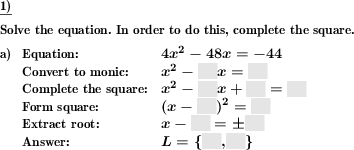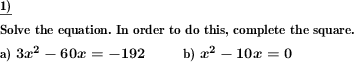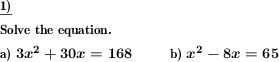Custom math worksheets at your fingertips# Details for problem "Quadratic formula: Complete square to solve equation"

Quickname: 2057

Junior High School, High School, Senior High School.

## Summary

To solve a quadratic equation, complete the square.

## Examples## Description

The solution set of a quadratic equation is to be determined. For this purpose the technique of completing the square is used.

If desired, the solution is described in the solution sheet in the steps

1. Convert to monic form
2. Complete the square
3. Combine like terms on right side
4. Introduce square
5. Extract root
6. Specify the solution set

is presented in detail. These steps can be presented in the problem definition as cloze text, the correct values must then be inserted.

In the problem definition the solution of a quadratic function is asked for. It can be set whether the way of solution via square completion should also be mentioned.

To simplify or complicate the problem, the degree of normalization can be changed. Thus it can be set whether the absolute element should be present (i.e. not equal to zero) or not, and whether the leading coefficient (of x^2) is equal to one or not. In the latter case, the conversion to monic form requires a division of the whole equation by the lead coefficient's value.

The number of problems is also adjustable.

Download free printable worksheets for this math problem here. The worksheet contains the problems only, the solution sheet includes the answers. Just click on the respective link.

•Worksheet 1Solution sheet with answers
•Worksheet 2Solution sheet with answers
•Worksheet 3Solution sheet with answers

If you can not see the solution sheets for download, they may be filtered out by an ad blocker that you may have installed. If this is the case, please allow ads for this page and reload the page. The solution sheets will then reappear.

• Do these sample worksheets do not really fit?
• Do you need more math worksheets, with a different level of difficulty?
• Would you like to combine different problems on a worksheet and adjust them to your needs?
• As a teacher, you can put together your own worksheets using the automatically generated math problems provided.
With a free initial credit, you can start creating your own math worksheets in a few minutes.

You can try it for free! Register here, to create custom worksheets now!

## Customization options for this problem

Parameter
Possible values
Number of problems
1, 2, 3, 4, 5, 6
Mention completion of square
Yes, No
Indicate steps
no, with solution, with problem&solution
Never, May, Always
Absolute value present
Never, May, Always

## Similar problems

Remark
Description
Complete the square to determine the vertex
To determine the vertex of a square function, convert it to vertex form by completing the square.